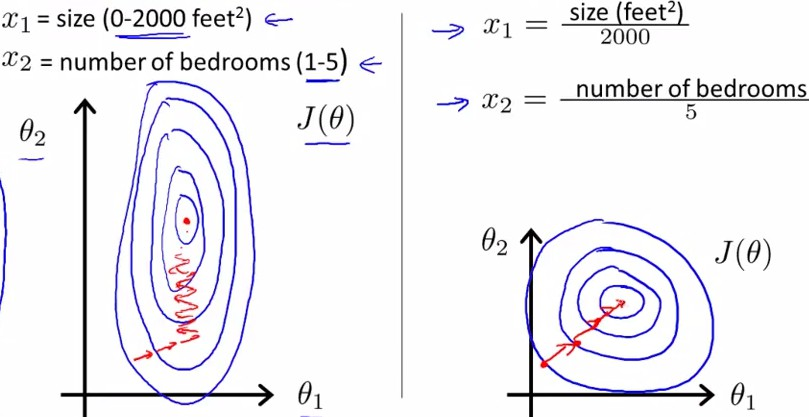数据预处理之归一化(标准化)[转]

1. 数据归一化及其应用

• 简单缩放
• 逐样本均值消减(也称为移除直流分量)
• 特征标准化(使数据集中所有特征都具有零均值和单位方差)

• 归一化后加快了梯度下降求最优解的速度;
• 归一化有可能提高精度;简单缩放 | min-max标准化(Min-max normalization) | 离差标准化

x ＝ (x - min)/(max - min)
max: 样本数据的最大值
min: 为样本数据的最小值

标准差标准化 | z-score 0均值标准化(zero-mean normalization)

x = (x - u)/σ
u: 所有样本数据的均值
σ: 为所有样本数据的标准差.

import numpy as np

X-=np.mean(X, axis=0) # X 的每一列都减去该列的均值
X/=numpy.std(X,axis=0) # 数据归一化

import numpy as np

X -= np.mean(X, axis=0) # 将数据零均值化
cov = np.dot(X.T, X)/X.shape  #计算协方差矩阵

# 去相关操作,
# 即通过将原始数据(零均值化后的数据)投影到特征基空间(eigenbasis)
U, S, V = np.linalg.svd(cov) # 计算数据协方差矩阵的奇异值SVD分解
Xrot = np.dot(X, U)  # 对数据去相关

# 白化处理，
# 即把特征基空间的数据除以每个维度的特征值来标准化尺度.
Xwhite = Xrot/np.sqrt(S+1e-5) #除以奇异值的平方根，1e-5 防止分母为 0.

PCA 白化的一个缺点是会增加数据中的噪声.

参考

 - 数据预处理
 - 数据的标准化
 - 为什么一些机器学习模型需要对数据进行归一化
 - 再谈机器学习中的归一化方法（Normalization Method）
 - sklearn scaler
 - sklearn scaler 2
 - 关于使用sklearn进行数据预处理 —— 归一化/标准化/正则化
 - 深度神经网络训练的必知技巧

Last modification：October 9th, 2018 at 09:31 am